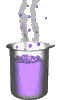Science class online
Chemistry and physics lessons

# Mechanics

Free online mechanics lesson for elementary school,  middle school and high school.Science class Chemistry Electricity Optics Mechanics Mechanics lessons Gravity - The solar system - motions of celestial bodies - Physical interactions - Effects of gravity - Weight of an object - Difference between weight and mass - Relationship between weight and mass  Energy - Energy basics - Potential energy - Kinetic energy - Mechanical energy - Translational Kinetic Energy - kinetic energy mass and speed

 _____________________________________________________   _____________________________________________________

## Gravity

### How speed and mass make kinetic energy vary

 1) mass Influences kinetic energy The relationship between Kinetic energy, mass and speed indicates that kinetic energy and mass are proportionals. This proportionality implies that if the mass is doubled, its kinetic energy is doubled too. More generally, if two objects move with the same speed the most massive one has the higher kinetic energy ______________________________________ ______________________________________ 2) Speed influences kinetic energy Kinetic energy isn't proportional to speed but to its square. If an object ( called object number one ) owns a kinetic energy KE1, a mass m and a speed v1, If a second object ( called object number two ) owns a kinetic energy KE2, a mass m and a speed v2 with speed v2 twice greater than speed v1 then: KE1 = 1 x m x v12             2 KE2 = 1 x m x v22             2 KE2 = 1 x m x (2 x v1 )2             2 KE2 = 1 x m x 22 x v12             2 KE2 = 1 x m x 4 x v12             2 KE2 =4 x ( 1 x m x v12)                    2 KE2 = 4 x  KE1 Kinetic energy of the second objet is four times greater than the first one. We could do the demonstration with v2 = 3 x v1: KE2 would be nine times ( 32 ) greater than KE1  We could also do the demonstration with v2 = 4 x v1: KE2 would be sixteen times ( 42 ) greater than KE1 etc ______________________________________ ______________________________________
____________________________________

____________________________________